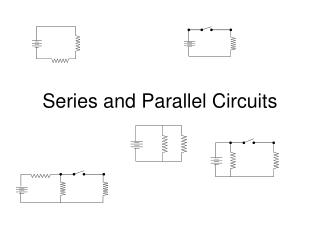Download PresentationSeries and Parallel Circuits

# Series and Parallel Circuits - PowerPoint PPT Presentation

Series and Parallel Circuits. Series Circuit. Series circuit - has only one path through which the electricity can flow. In the above diagram, the electricity flows through both loads. Current and Resistance in Series Circuits.I am the owner, or an agent authorized to act on behalf of the owner, of the copyrighted work described.
Download Presentation## Series and Parallel Circuits

Download Policy: Content on the Website is provided to you AS IS for your information and personal use and may not be sold / licensed / shared on other websites without getting consent from its author.While downloading, if for some reason you are not able to download a presentation, the publisher may have deleted the file from their server.

- - - - - - - - - - - - - - - - - - - - - - - - - - E N D - - - - - - - - - - - - - - - - - - - - - - - - - -
Presentation Transcript
1. Series and Parallel Circuits

2. Series Circuit • Series circuit - has only one path through which the electricity can flow. • In the above diagram, the electricity flows through both loads.

3. Current and Resistance in Series Circuits • For the series circuit the same current flows through both loads. • The loads can be added together to calculate the total load. • Rtot = R1 + R2, where Rtot is the total resistance, R1 is the resistance of one load, and R2 is the resistance of the other. • The total load (resistance) in a series circuit with “n” loads is the sum of the resistance of the “n” objects. Rtot = R1 + R2 + … + Rn.

4. Total Voltage in a Series Circuit • Ohm’s Law can be used to calculate the total voltage in a series circuit by calculating the sum of the voltage parts. • V = V1 + V2, where V is the total voltage (battery voltage), V1 is the voltage at the first load, and V2 is the voltage at the other load.

5. Parallel Circuits • A parallel circuit has multiple paths through which the electricity can flow. • In a parallel circuit, the current though one path may be different than the current through the other path.

6. Current in a Parallel Circuit • The total current in a parallel circuit is the sum of the two parts. • I = I1 + I2, where I is the total current, I1 is the current through one load, and I2 is the current through the other load. I1I2

7. Resistance in Parallel Circuits • Using Ohm’s Law you can derive a formula for the equivalent resistance of two resistors in parallel. • I1 = V/R1 • I2 = V/R2 • I = I1 + I2 = V/R1 + V/R2 • = (VR2 + VR1)/R1R2 = V(R2+R1)/R1R2 • Rtot = V/(V(R2+R1)/ R1R2 = R1R2/(R1+R2)